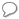xStochastic processOverview

In probability theory
Probability theory
Probability theory is the branch of mathematics concerned with analysis of random phenomena. The central objects of probability theory are random variables, stochastic processes, and events: mathematical abstractions of non-deterministic events or measured quantities that may either be single...

, a stochastic process (pron), or sometimes random process, is the counterpart to a deterministic process (or deterministic system). Instead of dealing with only one possible way the process might develop over time (as in the case, for example, of solutions of an ordinary differential equation
Ordinary differential equation
In mathematics, an ordinary differential equation is a relation that contains functions of only one independent variable, and one or more of their derivatives with respect to that variable....

), in a stochastic or random process there is some indeterminacy described by probability distributions. This means that even if the initial condition (or starting point) is known, there are many possibilities the process might go to, but some paths may be more probable and others less so.

In the simplest possible case (discrete time), a stochastic process amounts to a sequence of random variables known as a time series
Time series
In statistics, signal processing, econometrics and mathematical finance, a time series is a sequence of data points, measured typically at successive times spaced at uniform time intervals. Examples of time series are the daily closing value of the Dow Jones index or the annual flow volume of the...

(for example, see Markov chain
Markov chain
A Markov chain, named after Andrey Markov, is a mathematical system that undergoes transitions from one state to another, between a finite or countable number of possible states. It is a random process characterized as memoryless: the next state depends only on the current state and not on the...

).